﻿MultipleRegression.QR Method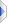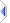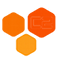MultipleRegressionQR Method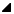Overload List
NameDescription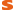QRT(MatrixT, MatrixT)
Find the model parameters β such that X*β with predictor X becomes as close to response Y as possible, with least squares residuals. Uses an orthogonal decomposition and is therefore more numerically stable than the normal equations but also slower.QRT(MatrixT, VectorT)
Find the model parameters β such that X*β with predictor X becomes as close to response Y as possible, with least squares residuals. Uses an orthogonal decomposition and is therefore more numerically stable than the normal equations but also slower.QRT(IEnumerableTupleT, T, Boolean)
Find the model parameters β such that their linear combination with all predictor-arrays in X become as close to their response in Y as possible, with least squares residuals. Uses an orthogonal decomposition and is therefore more numerically stable than the normal equations but also slower.QRT(T, T, Boolean)
Find the model parameters β such that their linear combination with all predictor-arrays in X become as close to their response in Y as possible, with least squares residuals. Uses an orthogonal decomposition and is therefore more numerically stable than the normal equations but also slower.
TopSee Also